# NCERT Solutions for Class 9 Maths Exercise 11.2## myCBSEguide App

CBSE, NCERT, JEE Main, NEET-UG, NDA, Exam Papers, Question Bank, NCERT Solutions, Exemplars, Revision Notes, Free Videos, MCQ Tests & more.

NCERT solutions for Class 9 Maths Constructions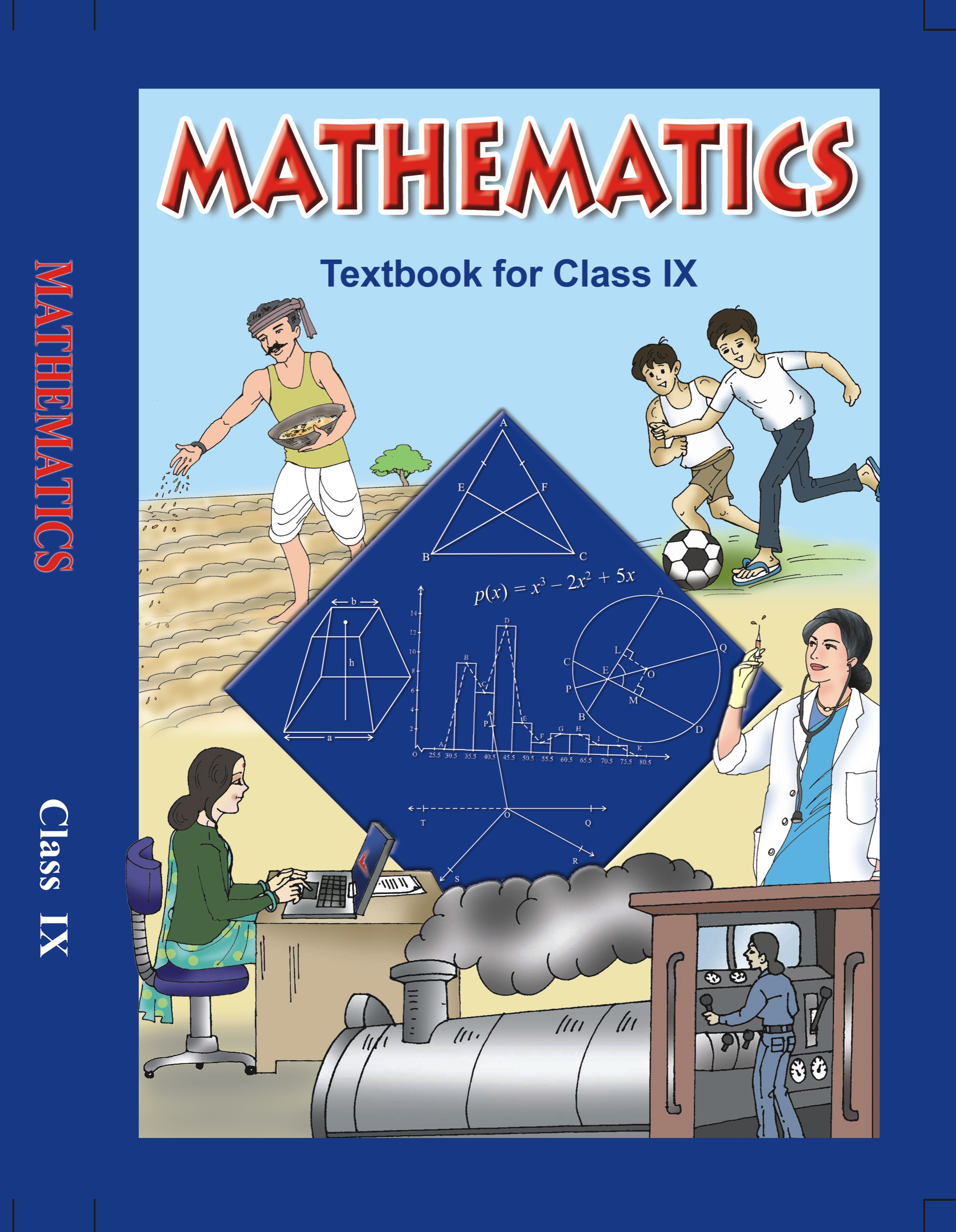## NCERT Solutions for Class 9 Mathematics Constructions

###### 1. Construct a triangle ABC in which BC = 7 cm,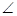B =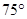and AB + AC = 13 cm.

Ans. Given : Base BC = 7 cm,B =and sum of two sides AB + AC = 13 cm.

To construct : A triangle ABC.

Steps of construction: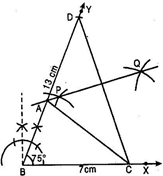(a) Draw a ray BX and cut off a line segment BC = 7 cm from it.

(b) At B, constructYBX =.

(c) With B as centre and radius = 13 cm (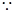AB + AC = 13 cm) draw an arc to meet BY at D.

(d) Join CD.

(e) Draw perpendicular bisector PQ of CD intersecting BD at A.

(f) Join AC.

Then ABC is required triangle.

Justification:

A lies on perpendicular bisector of CD.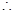AC = AD

And AB = BD -AD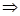AB = BD -ACAB + AC = BD = 13 cm

NCERT Solutions for Class 9 Maths Exercise 11.2

###### 2. Construct a triangle ABC in which BC = 8 cm,B =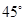and AB -AC = 3.5 cm.

Ans. Given: Base BC = 8 cm, One Base angleB =and AB -AC = 3.5 cm

To construct: A triangle ABC.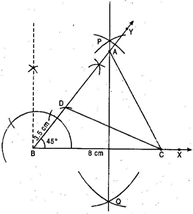Steps of construction:

(a) Draw a ray BX and cut off a line segment BC = 8 cm from it.

(b) CutYBC =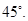(c) Cut off a line segment BD = 3.5 cm

(AB -AC = 3.5 cm) from BY.

(d) Join CD.

(e) Draw perpendicular bisector PQ of CD intersecting BY at a point A.

(f) Join AC.

Then ABC is the required triangle.

Justification:

A lies on the perpendicular bisector of CD.AD = ACBD = AB -AC = 3.5 cm

NCERT Solutions for Class 9 Maths Exercise 11.2

###### 3. Construct a triangle PQR in which QR = 6 cm,Q =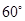and PR -PQ = 2 cm.

Ans. Given: Base QR = 6 cm, one base angleQ =and PR -PQ = 2 cm.

To construct: A triangle PQR.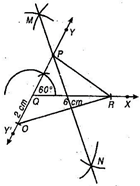Steps of construction:

(a) Draw a ray QX and cut off a line segment QR = 6 cm from it.

(b) Construct a ray QY making an angle ofwith QR and produce YQ to form a line YQY’.

(c) Cut off a line segment QO = 2 cm (PR -PQ = 2 cm) from QY’.

(d) Join OR.

(e) Draw perpendicular bisector MN of OR.

(f) Join PR.

Then PQR is the required triangle.

Justification:

P lies on perpendicular bisector of OR.PO = PRPQ + QO = PRQO = PR -PQ = 2 cm

NCERT Solutions for Class 9 Maths Exercise 11.2

###### 4. Construct a triangle XYZ in whichY =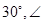Z =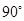and XY + YZ + ZX = 11 cm.

Ans. Given: Base anglesY =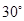andZ =and XY + YZ + ZX = 11 cm.

To construct: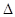XYZ

Steps of construction: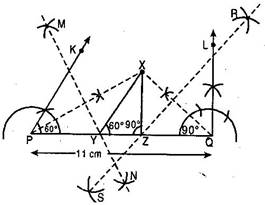(a) Draw a line segment PQ = 11 cm.

(b) DrawKPQ =andLQP =(c) Bisect theKPQ andLQP. Let these intersect at point X.

(d) Draw perpendicular bisectors, MN of PX and RS of XQ.

(e) Let MN intersects PQ at Y and RS intersects PQ at Z.

(f) Join XY and XZ.

Then XYZ is the required triangle.

Justification:

Y lies on perpendicular bisector MN of PX.PY = XY and similarly QZ = XZ

This gives XY + YZ + XZ = PY + YZ + QZ = PQ = 11 cm

AgainYXP =XPY [Since XY = PY]XYZ =YXP +XPY = 2XPY =KPQXYZ =Similarly,XZY =LQPXZY =NCERT Solutions for Class 9 Maths Exercise 11.2

###### 5. Construct a right triangle whose base is 12 cm and sum of its hypotenuse and other side is 18 cm.

Ans. Given: Base BC = 12 cm and AB + AC = 18 cm.

To construct: A right angled triangle ABC right angled at B.

Steps of construction: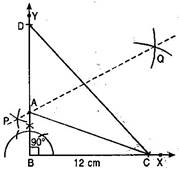(a) Draw a ray BX and cut off a line segment BC = 12 cm from it.

(b) Draw an angle XBY =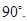(c) From BY cut off a line segment BD = 18 cm.

(d) Join CD.

(e) Draw the perpendicular bisector of CD intersecting BD at A.

(f) Join AC.

Then ABC is the required right angled triangle.

Justification:

A lies on the perpendicular bisector of CD.AC = AD

And then AB = BD -ADAB = BD -ACAB + AC = BD = 18 cm.

## NCERT Solutions for Class 9 Maths Exercise 11.2

NCERT Solutions for Class 9 Maths PDF (Download) Free from myCBSEguide app and myCBSEguide website. Ncert solution class 9 Maths includes text book solutions from Mathematics Book. NCERT Solutions for CBSE Class 9 Maths have total 15 chapters. 9 Maths NCERT Solutions in PDF for free Download on our website. Ncert Maths class 9 solutions PDF and Maths ncert class 9 PDF solutions with latest modifications and as per the latest CBSE syllabus are only available in myCBSEguide.

## CBSE app for Class 9

To download NCERT Solutions for Class 9 Maths, Computer Science, Home Science,Hindi ,English, Social Science do check myCBSEguide app or website. myCBSEguide provides sample papers with solution, test papers for chapter-wise practice, NCERT solutions, NCERT Exemplar solutions, quick revision notes for ready reference, CBSE guess papers and CBSE important question papers. Sample Paper all are made available through the best app for CBSE students and myCBSEguide website.### 7 thoughts on “NCERT Solutions for Class 9 Maths Exercise 11.2”

1. It was so helpful! Thank you very much

2. VERY NICE

3. Thank you so much for your help

4. These solutions are very clear to understand for every students.Thanks a lot for your kind and valuable help.I hope you will always help me in coming future.

5. very thanks

6. nice app it is so help full to me thanks very much

7. Thank u